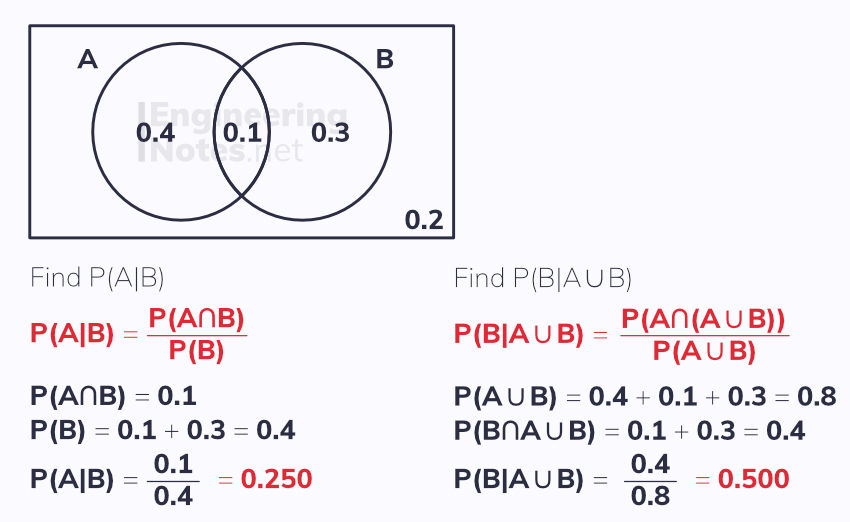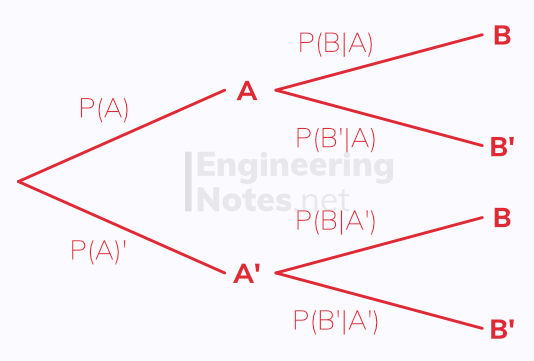top of page

#### Notes by Category University Engineering

Rate these notesNot a fanNot so goodGoodVery goodBrillRate these notes

# Probability

Probability is used to predict the likeliness of something happening. It is always given between 0 and 1.

• An experiment is a repeatable process that can have a number of outcomes

• An event is one single or multiple outcomes

• A sample space is the set of all possible outcomes

For two events, E₁ and E₂, with probabilities P₁ and P₂ respectively:

• To find the probability of either E₁ or E₂ happening, add the two probabilities, P = P₁ + P₂

• To find the probability of both E₁ and E₂ happening, multiply the two probabilities, P = P₁ x P₂

The sample space for rolling two fair six-sided dice and adding up the numbers that show would look like this:To work out the probability of getting a particular result, you count how many times the result occurs and divide by the total number, 36 (since 6² = 36). So to work out the probability of getting a 10, count the number of tens and divide by 36: 3/36 = 0.0833

## Conditional Probability

If the probability of an event is dependent on the outcome of the previous event, it is called conditional. Conditional probability is noted using a vertical line between the events:

The probability of B occurring, given that A has already occurred is given by P(B|A)

For two independent events:

P(A|B) = P(A|B') = P(A)

Experiments with conditional probability can be calculated using a two-way table/restricted sample space:## Venn Diagrams

A Venn diagram is used to represent events happening. The rectangle represents the sample space, and the subsets within it represent certain events. Set notation is used to describe events within a sample space:

A B represents the intersection
A ∪ B represents the union
Adding a dash, ', means the compliment, or "not"The addition formula is very useful:

P(A ∪ B) = P(A) + P(B) - P(A ∩ B)

### Mutually Exclusive Events

As you can see from the Venn diagrams, mutually exclusive events do not intersect. This means there is no overlap, so:

P(A or B) = P(A) + P(B)

### Independent Events

When one event has no effect on the other, the two events are described as independent. Therefore:

P(A ∪ B) = P(A) x P(B)

### Conditional Probability in Venn Diagrams

You can find conditional probability easily from Venn diagrams using the multiplication formula:

P(B|A) = P(B ∩ A) / P(A)## Tree Diagrams

Tree diagrams are used to show the outcomes of two or more events happening, one after the other.

For example, if there are 3 red tokens and 7 blue tokens in a bag, and two are chosen one after the other without replacement (the first is not put back into the bag), a tree diagram can model this:When you have worked out the probability of each branch, add them together - if they sum to 1, it is correct.

### Conditional Probability in tree diagrams

Tree diagrams show conditional probability in their second and third etc columns.The multiplication formula still applies:

P(B|A) = P(B ∩ A) / P(A)

See All
bottom of page